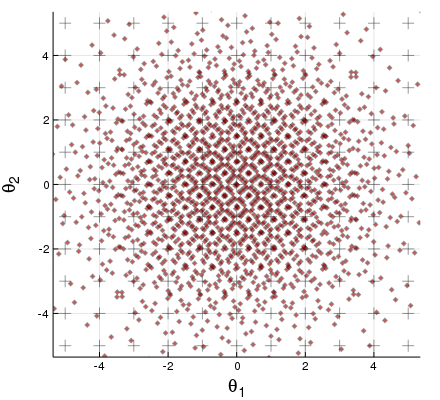dummy-link

0

0

0

3

# Weighted Arrays .jlThis simple package defines a `WeightedMatrix`, a struct with vector of weights corresponding to the columns of a matrix. By default the `weights(x)` add up to 1. The `array(x)` values may have a box constraint:

``````julia> Weighted(randn(3,5))
Weighted 3×5 Array{Float64,2}, of unclamped θ:
-0.264476   -1.83297      0.0669732  -0.340433  -1.87672
0.0461253  -0.330401     0.0215189   2.3129    -1.78839
0.461376    0.00486523  -0.819182   -1.43221   -0.855756
with normalised weights p(θ), 5-element Array{Float64,1}:
0.2  0.2  0.2  0.2  0.2

julia> Weighted(rand(2,4), ones(4), 0, 1)
Weighted 2×4 Array{Float64,2}, clamped 0.0 ≦ θ ≦ 1.0:
0.7842    0.257179  0.483388  0.780996
0.138967  0.748165  0.387104  0.167825
with normalised weights p(θ), 4-element Array{Float64,1}:
0.25  0.25  0.25  0.25
``````

These examples are roughly `wrandn(3,5)` and `wrand(2,4)`, there are also sub-random `sobol(3,7)` and regular `wgrid(2, 0:0.1:1)`. Their values are mutable, `clamp!(x)` will enforce the box constraint, and `normalise!(x)` (with an s) the weights.

They are not subtypes of `AbstractArray`, but many functions will work. For instance `x[1:2, :]` keeps only the first two rows (and the weights), `hcat(x,y)` will concatenate the weights, and `mapslices(f,x)` will act with `f` on columns & then restore weights. `sort(x)` re-arranges columns to order by the weights, `sortcols(x)` orders by the array instead, `unique(x)` will accumulate the weights of identical columns. A few functions like `log(x)` and `tanh(x)` act element-wise but update the box constraints appropriately.

Most of this will work for any N-dimensional Array, not just a Matrix. The weights then belong to the last dimension.Plot recipes are defined, in which the area of points indicating weight. The example shown is a grid plus a bivariate sub-random normal distribution:

``````julia> using Plots

julia> plot(wgrid(2, -5:5), m=:+)

julia> plot!(soboln(2, 2000), m=:diamond, c=:red)
``````

With more than three rows e.g. `plot(wrandn(4,50))`, it will plot the first two principal components (and attempt to scale these correctly). There is a function `pplot(x)` which saves the PCA function (see help for `wPCA(x)`) in a global variable, so that `pplot!(t)` can add more points on the same axes.

08/17/2018

5 months ago

16 commits